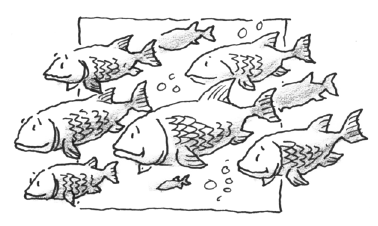### Home > APCALC > Chapter 11 > Lesson 11.2.2 > Problem11-59

11-59.

The population $P$ of a certain species of fish grows at a rate of $\frac { d P } { d t }= 0.01P(100 − P)$ fish per year. When time $t = 0$, the population is $50$ fish. What is the population after two years?$\frac{100dP}{P(100-P)}=dt$

$\int\frac{100dP}{P(100-P)}=\int dt$

$\frac{100}{P(100-P)}=\frac{1}{P}+\frac{1}{100-P}$

$\ln|P|-\ln|100-P|=t+C$

$\ln\Big|\frac{P}{100-P}\Big|=t+C$

$Ce^t=\frac{P}{100-P}$

When $t = 0$, $P = 50$. What is the value of $C$?

Use your value of $C$ in your equation to determine the value of $P$ when $t = 2$.